# Maharashtra Board Class 12 Economics Important Questions Chapter 3A Demand Analysis

Balbharti Maharashtra State Board Class 12 Economics Important Questions Chapter 3A Demand Analysis Important Questions and Answers.

## Maharashtra State Board 12th Economics Important Questions Chapter 3A Demand Analysis

1. A. Choose the correct option:

Question 1.
The demand curve slopes downward.
(a) Inverse relationship between price quantity demanded
(b) from left to right
(c) from right to left
(d) Direct relationship quantity demanded
Options :
(1) a,b and c
(2) a, b, c and d
(3) a and b
(4) a and d
(3) a and b

Question 2.
Demand for a commodity from an individual consumer.
(a) Direct demand
(b) Market demand
(c) Individual demand
(d) Increase in demand
Options:
(1) a
(2) b
(3) c
(4) d
(3) cQuestion 3.
Demand for a commodity from different consumer at different prices.
(a) Decrease in demand
(b) Contraction in demand
(c) Individual demand
(d) Market demand
Options:
(1) c
(2) b
(3) a
(4) d
(4) d

Question 4.
Direct demand.
(a) Vegetable
(b) Milk
(c) Land
(d) Cloth
Options :
(1) a,b and c
(2) a, b and d
(3) b, c and d
(4) c
(2) a, b and d

Question 5.
Indirect demand.
(a) Machinery
(b) Sugar
(c) Labour
(d) Capital
Options :
(1) a, b, c and d
(2) a, b and c
(3) a, b and d
(4) a, c and d
(4) a, c and d

Question 6.
Joint demand for i tea.
(a) Sugar
(b) Milk
(c) Tea powder
(d) Rice
Options :
(1) a,b and c
(2) b and d
(3) a,b and d
(4) b, c and d
(1) a,b and cQuestion 7.
Determinant of Demand.
(a) Price
(b) Population
(c) Income
Options :
(1) a and d
(2) a, c and d
(3) a, b, c and d
(4) c and d
(3) a, b, c and d

Question 8.
Composite demand.
(a) Electricity
(b) Water
(c) Steel
(d) Xerox machine
(1) a, b and d
(2) a, b and c
(3) a, b and d
(4) a and d
(2) a, b and c

Question 9.
Competitive demand.
(a) Thums-up
(b) Pepsi
(c) Buttermilk
(d) Coco-cola
Options:
(1) a, b and c
(2) a, c and d
(3) a, b and d
(4) c and d
(3) a, b and d

Question 10.
The law of demand was introduced by neo-classical economist.
(a) Alfred Marshall
(c) Ragnar Frisch
(d) Samuelson
Options:
(1) e
(2) b
(3) d
(4) a
(4) a

Question 11.
Assumptions for the law of Demand.
(a) Constant Income
(b) No change in taxation policy
(d) Stable population
Options :
(1) a, b and d
(2) a, b and c
(3) a and d
(4) only d
(1) a, b and dQuestion 12.
Exceptions to the law of Demand.
(a) Inferior goods
(b) Jewellery
(c) Habitual goods
(d) Price of substitute goods
Options :
(1) a, b and d
(2) a, b and c
(3) only d
(4) a, c and d
(2) a, b and c

Question 13.
Variation in Demand.
(a) Change in Price
(b) Change in quantity demanded
(c) Change in other factors
(d) No change in other factors
Options :
(1) a, b and c
(2) a, b and d
(3) c and d
(4) only a and b
(2) a, b and d

Question 14.
Expansion of Demand.
(a) Fall in price
(b) Rise in quantity demanded
(c) No change in income
(d) Change in population
Options :
(1) only c
(2) a, b and c
(3) a, b and d
(4) b, c, and d
(2) a, b and c

Question 15.
Change in Demand.
(a) Constant price
(b) Change in demand
(c) Changes in other factors
(d) Increase and Decrease in demand
Options :
(1) a and b
(2) c and d
(3) a, b, c and d
(4) None of these
(3) a, b, c and d

Question 16.
Individual demand.
(a) Micro economics
(b) Slicing method
(c) Lumping method
(d) Study of individual unit
Options :
(1) a, b and d
(2) b and c
(3) b, c and d
(4) only a
(1) a, b and d

Question 17.
Market demand.
(a) Aggregate demand
(b) Macro economics
(c) Micro economics
(d) Lumping method
Options :
(1) a, b and d
(2) b, c and d
(3) b and c
(4) only c
(1) a, b and dQuestion 18.
Decrease in demand.
(a) Favourable changes in other factors
(b) Unfavourable changes in other factors
(c) Decrease in quantity demanded
(d) Changes in income of the consumer
Options :
(1) b, c and d
(2) a, b and c
(3) a and b
(4) a, c and d
(1) b, c and d

(B) Complete the Correlation.

(1) Individual consumer ……………… :: Total of all the individual: Market demand
(2) Labour : Indirect demand:: Clothes : ………………
(3) Joint demand : Pencil and Paper :: ……………… Electricity
(4) Complementary demand : Car and Petrol:: ………………: Pepsi and Coke
(5) Constant level of Income : Assumption : : Prestige goods : ………………
(6) Expansion in demand : Downward movement on the demand curve :: ………………: Upward movement on the demand curve
(7) Change in demand : Increase in Demand :: ………………: Extension in demand
(8) Increase in demand : Favourable changes in other factors :: Decrease in demand : ………………
(9) Fall in Price : ……………… :: Rise in Price : Quantity demanded falls
(10) ……………… : Tea :: Composite demand : Milk
(1) Individual demand
(2) Direct demand
(3) Composite demand
(4) Competitive demand
(5) Exception
(6) Contraction in demand
(7) Variation in demand
(8) Unfavourable changes in other factors
(9) Quantity demanded rises
(10) Joint demand

(C) Give economic terms.

(6) Desire + willingness to purchase + Ability to pay.
(7) The demand by a single consumer in a market.
(8) The demand by all consumer in a market.
(9) Other factors remaining constant, a fall in demand due to a rise in price.
(10) Other factors remaining constant, a rise in demand due to a fall in price.
(11) To satisfying a want of sewing, needle and thread is required.
(12) A rise in demand due to changes in other factors, price remaining constant.
(13) Fall in demand due to changes in other factors, price remaining constant.
(14) Goods of low quality are considered as inferior goods.
(15) The demand for commodities to satisfy the human wants directly.
(16) The demand for factors of production like land, labour, etc.
(17) Two or more goods are demanded at a time to satisfy one want.
(6) Demand
(7) Individual Demand
(8) Market Demand
(9) Contraction in Demand
(10) Expansion in Demand
(11) Complementary or Joint Demand
(12) Increase in Demand
(13) Decrease in Demand
(14) Giffen’s Goods
(15) Direct Demand
(16) Indirect Demand
(17) Joint or Complementary Demand

(D) Find the o’dd word out.

(1) Desire, Ability to pay, Uselessness, \ Willingness to purchase
(2) Pepsi, Limca, Coffee, Coke
(3) Cloth, Sugar, Fertilizer, Milk
(4) Land, Capital, Raw material, Flowers
(5) Tea and Sugar, Pen and Pencil, Car and Petrol, Mobile phones and Sim cards
(6) Indirect demand, Individual demand, Composite demand, Competitive demand
(7) Consumer demand, Complementary demand, Composite demand, Competitive demand
(8) Tea – Coffee, Car – Fuel, Thums-up – Pepsi, Rice – Wheat
(9) Coffee powder, Chilli powder, Milk, Sug >r
(10) Gold, Diamond, Platinum, Aluminium
(11) Sony, Samsung, Onida, Karbonn
(1) Uselessness
(2) Coffee
(3) Fertilizer
(4) Flowers
(5) Pen and Pencil
(6) Individual demand
(7) Consumer demand
(8) Car – Fuel
(9) Chilli powder
(10) Aluminium
(11) Karbonn(E) Complete the following statements.

(1) The willingness to have something is called …………….
(2) Desire, willingness to purchase and ability to pay are the three necessary conditions for …………….
(3) The total quantities of a commodity demanded by a particular buyer is ……………..
(4) The sum of the total quantities of a commodity demanded by all buyers in a market is ……………..
(5) Commodities and services satisfying the human wants directly is known as ……………..
(6) The purchasing power of the consumer depends on ……………..
(7) One commodity can be put to several uses, it is known as ……………..
(8) Marshall’s law of demand describes the functional relationship between ……………..
(9) Inferior goods like cheap bread, vegetable ghee, etc., is known as ……………..
(10) Expensive goods such as diamonds, luxury cars are known as ……………..
(11) When demand changes due to changes in price, it is known as ………………
(12) A rise in demand caused by favourable changes in other factors at same price is called ………………
(1) desire
(2) demand
(3) individual demand
(4) market demand
(5) direct demand
(6) ability to pay
(7) composite demand
(8) Demand and Price
(9) Giffen goods
(10) Prestige goods
(11) variation in demand
(12) increase in demand

(F) Choose the wrong pair :

I.

 Group ‘A’ Group ‘B’ (1) Individual demand Particular consumer (2) Complementary demand Pen – Ink (3) Competitive demand Milk – Sugar (4) Indirect demand Labour

Wrong pair : Competitive demand – Milk- 5 Sugar

II.

 Group ‘A’ Group ‘B’ (1) Expansion of demand Other factors remaining constant (2) Law of demand Slopes downwards from left to right (3) Market demand Demand of all consumers (4) Direct demand Factors of production

Wrong pair : Direct demand – Factors production

III.

 Group ‘A’ Group ‘B’ (i) Inferior goods Giffen goods (2) Prestige goods Luxury goods (3) Expanasion of demand Fall in price (4) Increase in demand Unfavourable changes in other factors

Wrong pair : Increase in demand Unfavourable changes in other factors

IV.

 Group ‘A’ Group ‘B’ (1) Sir Robert Giffen England (2) Increase in real income Decrease in demand (3) Inferior goods Bread (4) Superior goods Meat

Wrong pair : Increase in real income Decrease in demand

V.

 Group ‘A’ Group ‘B’ (1) Individual demand Individual consumer (2) Joint demand Tea-coffee (3) Variation in demand Other factors remaining constant (4) Decrease in demand Price remains constant

(2) Joint demand Tea-coffee

(G) Assertion and Reasoning

Question 1.
Assertion (A) – Individual demand refers to the quantity of a commodity demanded by particular consumer.
Reasoning (R) – Market demand refers to the total demand for commodity from all the consumers.
(i) (A) is true but (R) is false.
(ii) (A) is false but (R) is true. .
(iii) Both (A) and (R) is true and (R) is the correct explanation of (A).
(iv) Both (A) and (R) is true but (R) is not the correct explanation of (A).
(iv) Both (A) and (R) is true but (R) is not the correct explanation of (A).

Question 2.
Assertion (A) – Marginal utility goes on diminishing with an increase in the stock of ) a commodity. I
Reasoning (R) – Purchasing power of a consumer increases when the price of a commodity increases. 5-
(i) (A) is true but (R) is false.
(ii) (A) is false but (R) is true.
(iii) Both (A) and (R) is true and (R) is the correct l explanation of (A).
(iv) Both (A) and (R) is true but (R) is not the ( correct explanation of (A).
(i) (A) is true but (R) is false.

Question 3.
Assertion (A) – Indirect demand refers to the demand for goods and services which are needed for further production.
Reasoning (R) – All factors of production have indirect or derived demand.
(i) (A) is true but (R) is false.
(ii) (A) is false but (R) is true.
(iii) Both (A) and (R) is true and (R) is the correct explanation of (A).
(iv) Both (A) and (R) is true but (R) is not the correct explanation of (A).
(iii) Both (A) and (R) is true and (R) is the correct explanation of (A).

Question 4.
Assertion (A) – When the population size is larger, demand for commodity will be less.
Reasoning (R) – Change in the price of one commodity would also affect the demand of substitute goods.
(i) (A) is true but (R) is false.
(ii) (A) is false but (R) is true.
(iii) Both (A) and (R) is true and (R) is the correct explanation of (A).
(iv) Both (A) and (R) is true but (R) is not the correct explanation of (A).
(ii) (A) is false but (R) is true.Question 5.
Assertion (A) – Demand curve slopes downwards from left to right.
Reasoning (R) – The price of a commodity falls, quantity demanded rises and when S price of commodity rises, quantity demanded falls.
(i) (A) is true but (R) is false.
(ii) (A) is false but (R) is true.
(iii) Both (A) and (R) is true and (R) is the correct explanation of (A).
(iv) Both (A) and (R) is True but (R) is not the correct explanation of (A).
(iii) Both (A) and (R) is true and (R) is the correct explanation of (A).

Question 6.
Assertion (A) – If consumer can anticipate the future price of a commodity then it will affect the present demand of a commodity.
Reasoning (R) – Ram buy’s less mangoes in anticipation of getting it at cheaper rate in further date.
(i) (A) is true but (R) is false.
(ii) (A) is false but (R) is true.
(iii) Both (A) and (R) is true and (R) is the correct explanation of (A).
(iv) Both (A) and (R) is true but (R) is not the correct explanation of (A).
(iii) Both (A) and (R) is true and (R) is the correct explanation of (A).

Question 7.
Assertion (A) – Variations in demand refers to change in quantity demanded due to change in price alone and other factors remain constant.
Reasoning (R) – Increase in demand and decrease in demand are the types of variations in demand.
(i) (A) is true but (R) is false.
(ii) (A) is false but (R) is true.
(iii) Both (A) and (R) is true and (R) is the correct explanation of (A).
(iv) Both (A) and (R) is true but (R) is not the correct explanation of (A).
(i) (A) is true but (R) is false.

Question 8.
Assertion (A) – Increase in demand refers rise in quantity demanded due to favourable changes in other factors and price remains constant.
Reasoning (R) – Decrease in demand refers to fall in quantity demand due to unfavourable changes in other factors and price remains constant.
(i) (A) is true but (R) is false.
(ii) (A) is false but (R) is true.
(iii) Both (A) and (R) is true and (R) is the correct explanation of (A).
(iv) Both (A) and (R) is True but (R) is not the ) correct explanation of (A).
(iii) Both (A) and (R) is true and (R) is the correct explanation of (A).

(H) Choose the correct pair :

I.

 Group ‘A’ Group ‘B’ (1) Joint Demand (a) Luxury car (2) Demand and price (b) Exception to the law of demand (3) Giffen’s goods (c) Inverse relationship (4) Prestige goods (d) Several commodities (e) Vegetables

(1)-(d), (2)-(c), (3)-(b), (4)-(a).

II.

 Group ‘A’ Group ‘B’ (1) Factors of production (a) Change in price alone (2) Inferior goods (b) Change in other factors (3) Pen and Pencil (c) Substitute goods (4) Change in Demand (d) Giffen’s goods (e) Indirect demand

(1) – (e), (2) – (d), (3) – (c), (4) – (b).

III.

 Group ‘A’ Group ‘B’ (1) Contraction in demand (a) Less is demanded at a same price (2) Decrease in demand (b) Complementary goods (3) Demand curve (c) Substitute goods (4) Tea and Coffee (d) Less is demanded at a higher price (e) Slopes downwards from left to right

(1)-(d), (2)-(a), (3)-(e), (4) – (c).IV.

 Group ‘A’ Group ‘B’ (1) Demand (a) A new demand curve (2) Variation in Demand (b) Same demand curve (3) Extension of demand (c) Ability and willingness to pay (4) Increase in demand (d) Change in price alone (e) Distribution of income

(1)-(c), (2)-(d), (3)-(b), (4)-(a).

V.

 Group ‘A’ Group ‘B’ (1) Demand (a) Exceptional demand (2) Prestige goods (b) Prof. Marshall (3) Joint demand (c) Demand curve shift to right (4) Demand increases (d) Complementary demand (e) A higher price

(1)-(b), (2)-(a), (3)-(d), (4)-(c).

2.[A] Identify and explain the concept from given illustrations.

Question 1.
Ramesh has bought 1kg of Tea powder instead of expensive coffee powder.
Concept: Competitive Demand
Explanation : Competitive Demand refers to demand for those goods which substitute for each other.
Change in the price of one commodity can affect the demand for substitute goods. Ramesh has found price of coffee at very high level, thus he bought tea powder.

Question 2.
Since Manoj’s salary has increased he has bought 5 kgs of rice instead of 3 kgs rice which he buys normally.
Concept: Increase in demand
Explanation : Increase in demand refers to change in quantity demanded due to favourable changes in other factors like tastes, income, etc., and price remains constant.
Manoj has got increment this month and hence he has bought more quantity of rice at same price.

Question 3.
Due to increase in population of Mumbai, demand for electricity is rising day by day.
Concept: Composite demand
Explanation : Composite demand refers to the demand for a commodity which can be put to several uses.
Demand for electricity is rising due to more industries, increase in household consumption etc.
Due to urbanization and industrialization many people are getting migrated to Mumbai and thus the consumption of electricity in increasing day by day.

Question 4.
Pari has bought most desirable Apple iphone 11 pro.
Concept: Demand
Explanation : In economics demand means a desire which is backed by willingness and ability to pay.
It was Part’s most favourite phone, thus she spend whatever price for it was and bought the phone.

Question 5.
Whenever there is decrease in price of gold, demand for gold rises.
Concept : Expansion or Extension of Demand
Explanation : Expansion of Demand refers to rise in quantity demanded due to fall in price alone while other factors like tastes, income, etc., remain unchanged.
There is general tendency of consumer to buy more whenever price falls.
Gold is most preferred investment of general public thus it is most demanded with fall in price.

(B) Distinguish between.

Question 1.
Individual Demand and Market Demand.
Individual Demand:

1. Individual demand refers to total quantities of a commodity demanded by an individual or household at a given price during a period of time.
2. It is basically micro or narrow concept and not very useful in framing business, production and price policies.
3. Individual demand depend on the price of goods, disposable income credit facilities, taste, preference, etc.
4. It can be presented with the help of individual demand schedule and individual demand curve.

Market Demand :

1. Market demand refers to the total quantities of a commodity demanded by all the individuals in the market at a given price, during a given period of time.
2. It is a macro or broader concept and is very useful in framing, business, production and price policies. Even the law of demand is based on market demand.
3. Market demand depends on size of population, social customs, distribution of income, etc.
4. It can be presented with the help of market demand schedule and market demand curve.

Question 4.
Inferior Goods or Giffen’s Goods and Normal Goods.
Inferior Goods or Giffen’s Goods:

1. Giffen’s goods refers to inferior goods like cheap quality rice, vegetable ghee, bread, etc. purchased by poor people.
2. The demand for inferior goods falls when prices falls.
3. The demand curve for Giffen’s goods is upward sloping from left to right as shown below.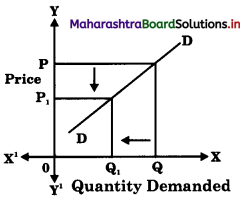4. Giffen’s goods are exceptions to the law of demand. As price and demand are directly related.

Normal Goods:

1. Normal goods are those goods which are better in quality and purchased by higher income group like rice, sugar, clothes.
2. The demand for normal goods falls when price rises.
3. The demand curve for normal goods is downwards sloping from left to right as shown below.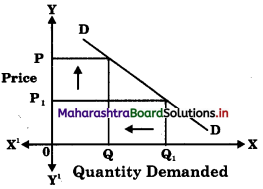4. Normal goods represent the law of demand.
As price and demand are inversely related.Question 5.
Normal Demand Curve and Exceptional Demand Curve.
Normal Demand Curve:

1. Normal demand curve represents the law of demand, which states that demand is inversely related to price.
2. The normal demand curve slopes downwards from left to right.
3. Normal goods like rice, wheat, clothes have normal demand curve.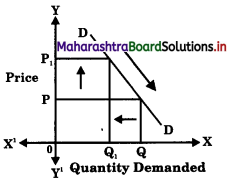Exceptional Demand Curve:

1. Exceptional demand curve goes against the law of demand. It represents a direct relationship between price and demand.
2. Exceptional demand curve slopes upwards from left to right.
3. Giffen’s goods, prestige goods, branded goods, habituated goods, like cigarette have exceptional demand curve.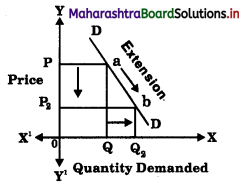Question 6.
Direct Demand and Derived Demand / Indirect Demand
Direct Demand:

1. Demand for consumer goods is direct because it satisfies our wants directly.
2. Demand for consumers goods are directly for consumption.
3. Direct demand comes from consumers or household sector.
4. Direct demand depends on price, income, taste, habits, etc. of consumers.
5. E.g. all consumer goods like books, furniture, T.V., tea, etc. have direct demand.

Derived Demand / Indirect Demand:

1. Demand for factors of production like land, labour, capital is derived as they satisfy our wants indirectly
2. Demand for factors of production are for further production.
3. Derived demand comes from producers or firms.
4. Derived demand depends on demand for final goods and services.
5. E.g. when demand for cotton shirt will increase, demand for raw cotton increases.

Question 7.
Extension I Expansion of Demand and Increase in Demand.
Extension / Expansion of Demand:

1. Extension of demand refers to a rise in demand due to fall in price. Other factors remaining constant.
2. It is a case of variation in demand.
3. Extension in demand takes place due to a fall in price, other things remaining constant.
4. When there is extension in demand the demand curve moves downward on the same demand curve.Increase in Demand:

1. Increase in demand takes place when more quantity is demanded at the same price.
2. It is a case of changes in demand.
3. Increase in demand takes place due to change in other factors, price remaining constant such as change in income, taste, habits, population, etc.
4. When there is a increase in demand the demand curve shifts to the right of the otiginal demand curve.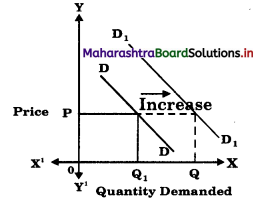Question 8.
Contraction of Demand and Decrease in Demand
Contraction of Demand

1. Contraction of demand means fall in demand due to a rise in price alone, other factors remaining constant.
2. Contraction of demand is a case of variation of demand.
3. Contraction in demand takes place due to rise in price alone.
4. It is shown by an upward movement along the same demand curve.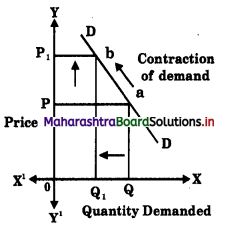Decrease in Demand

1. Decrease in demand means when less is demanded at the same price or same quantity demanded at a lower price.
2. Decrease in demand is a case of changes in demand.
3. Decrease in demand is due to –
(a) decrease in income
(b) decrease in price of substitute
(c) increase in price of complementary goods
(d) decrease in population, etc.
4. A decrease in demand is shown by a shift in demand curve to the left of the original demand curve.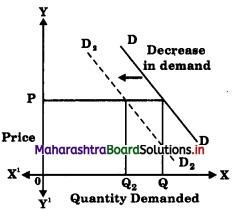Question 9.
Variation in Demand and Chañges in Di
Variation in Demand :

1. Variation in demand refers to changes in quantity demanded due to changes in price, other things remaining constant.
2. There are two types of variation in demand –
(a) Expansion or Extension in demand.
(b) Contraction in demand.
3. When price rises, quantity demanded falls, it is known as contraction and when price falls quantity demanded rises, it is known as expansion.
4. Variation in demand are shown by a movement along the same demand curve. Upward movement indicates contraction and downward movement indicates expansion.Changes in Demand:

1. Changes in demand refers to changes in quantity demanded due to changes in factor other than price like change in income of consumer, size of population, change in fashion, change in price of substitute, etc.
2. There are two types of changes in demand –
(a) Increase in demand
(b) Decrease in demand
3. Increase in demand means when more quantity is demanded at the same price and decrease in demand means when less quantity is demanded at a same price.
4. Changes in demand are shown by shift in demand curve. Increase in demand is shown by a shift in demand curve to right side and decrease in demand is shown by a shift to the left side.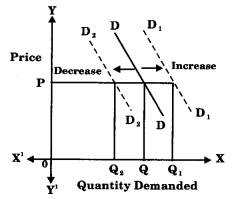Question 10.
Joint Demand and Composite Demand.
Joint Demand:

1. When two or more goods are demanded jointly to satisfy a single want, it is called joint or complementary demand.
2. E.g. of joint demand are car and petrol, ink cartridge and printers, mobile and sim card.
3. A rise in demand for one product will lead to a rise in the demand for other and vice versa.
4. In case of joint demand an increase in price of one good will reduce the demand for its joint product i.e. a rise in price of petrol will reduce the demand for car.
5. The demand for joint products is inelastic.

Composite Demand:

1. When goods are demanded for several uses, it is the case of composite demand.
2. E.g. of composite demand are for electricity, coal, steel, sugar, etc.
3. A change in demand for one use will affect the supply in other use.
4. In case of composite demand, an increase in the price of product will reduce its demand for different uses. Electricity / coal will be put to only important uses when its price rises.
5. The demand for composite commodities is elastic.

Question 11.
Complementary Demand or Joint Demand and Competitive Demand.
Complementary Demand or Joint Demand:

1. When two or more goods are demanded jointly to satisfy one want, it is called complementary or joint demand.
2. E.g. bread and butter, car and petrol, mobile and sim card.
3. A rise in demand for one product will lead to a rise in the demand for the other and vice versa.
4. The cross elasticity of demand is negative.
5. When price of one commodity rises the demand for the other commodity i.e. its joint product falls. When price of car falls the demand for petrol rises.
6. The demand curve has a negative slope.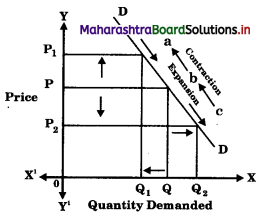Competitive Demand Demand:

1. When two goods are close substitutes i.e. the demand for one competes with that of the other it is a case of competitive demand.
2. E.g. tea or coffee, pepsi or coke, petrol or diesel, Lux or Dove.
3. Rise in demand for one product will lead to a fall in the demand for other and vice versa.
4. The cross elasticity of demand is positive.
5. When the price of one commodity rises, the demand for the substitute commodity rises. When price of tea rise, the demand for coffee increases.
6. The demand curve has a positive slope.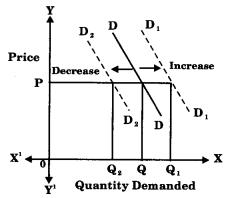Question 12.
Demand and Aggregate Demand.
Demand:

1. Demand is a micro concept.
2. It refers to that quantity of a commodity which a person is ready to buy at a particular price and during a specific period of time.

Aggregate Demand:

1. Aggregate demand is a macro concept.
2. It refers to the total amount of sales proceeds which an entrepreneur actually expects from the sale of output produced at a given level of employment during the year.

Question 1.
Write short notes on types of demand.
Types of Demand:

1. Direct Demand : When a commodity satisfies the want of a consumer directly, it is said to have direct demand. Demand for consumer goods like food, clothes, sugar, vegetables, milk, etc.
2. Indirect Demand : It refers to demand for goods which are required for further production. It is also called as derived demand. Demand for factors of production like land, labour, capital, etc.
3. Joint or Complementary Demand : When two or more goods are demanded at a same time to satisfy single want, it is known as Joint or Complementary Demand. E.g. car and fuel, pen and ink, mobile phone and sim card, etc.
4. Composite Demand : When one commodity can be utilize for several needs or multiple uses, it is known as composite demand. E.g. electricity, steel, coal, etc.
5. Competitive Demand : It is demand for those goods which are substitute for each other. E.g. tea or coffee, sugar or jaggery, pepsi or Thumsup, etc.Question 2.
Explain Market Demand schedule with a suitable diagram.
It refers to total demand for a commodity from all the consumers. It is total quantity of commodity demanded by different consumers at different prices during a given period of time.
Market Demand Schedule is a tabular representation of various quantities of a commodity demanded by different consumers at different prices during a given period of time.
This can be explained with the help of following schedule-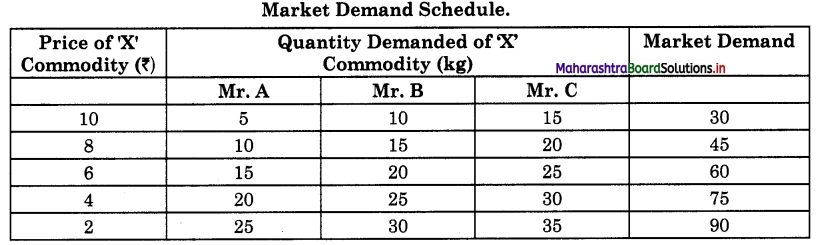The above market demand schedule shows an inverse relationship between price and market demanded of commodity ‘X’. At a high price of ₹10 per kg of commodity ‘X’, the market demand is only 30 kg and at a lower price of market demand rises to 90 kg of commodity ‘X’.

Market Demand Curve.
It is a graphical representation of market demand schedule X-axis represents quantity demanded (Market Demand) and Y-axis represents the price of the commodity. When the above market demand schedule is plotted on the graph, we derive the market demand curve ‘DD’, which slopes downward from left to right indicating inverse relationship between price and quantity demanded.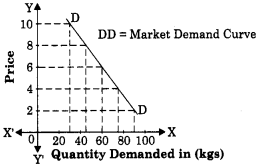Question 3.
Explain the concept of change in j demand.
Change in demand refers to change in s quantity demanded due to change in other factors of demand and price remains constant.
There are two types of changes in demand. They are : (1) Increase in demand (2) Decrease in demand.

(1) Increase in demand : It refers to rise in quantity demanded due to favourable changes in other factors like tastes, income, etc. and price remains constant.
Demand curve shifts to the right hand side of the original demand curve.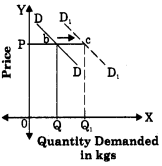In the above diagram, DD is the demand curve which is showing downward movement on the same demand curve from point ‘b’ to point ‘c’ which indicates an expansion of demand.

(2) Decrease in demand : It refers to fall in quantity demanded due to unfavourable changes in other factors like tastes, income, etc. and price remains constant. Demand curve shift to the left hand side of the original demand curve.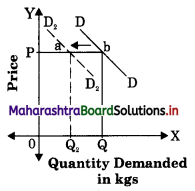In the above diagram DD is the original demand curve. D2 D2 demand curve indicates, decrease in demand by shifting towards the left hand side of original demand curve from point ‘b’ to point ‘a’.

Question 4.
Explain the assumptions to the Law of Demand.
Assumptions to the Law of Demand:

• Prices of Substitute goods remain constant : The price of substitute goods should remain unchanged, as change in the price will affect the demand for the commodity.
• Prices of Complementary goods remains constant : A change in the price of one good will affect the demand for other, thus the prices of complementary goods should remain unchanged.
• No Expectation about future changes in prices : The consumers do not expect any significance rise or fall in the future prices.
• No change in size of Population : The size of population, gender ratio and age composition are assumed to remain constant. As such changes are sure to affect demand.
• No change in Taxation Policy : The level of direct and indirect tax imposed by the government on the income and goods should remain constant.
• Constant Level of Income : Consumer’s income must remain unchanged because if income increases, consumer may buy more even at a higher price not following the law of demand.
• No Change in Tastes, Habits,
Preference, Fashions, etc. : If the taste changes then the consumers preference will also change which will affect the demand. When commodities are out of fashion, then demand will be low even at a lower price.Question 5.
What is meant by extension and contraction of demand?
Variations in demand refers to change in quantity demanded for a commodity due to change in price alone and other factors remaining constant.
There are two types of variations in demand.
They are (1) Expansion or Extension of demand (2) Contraction of Demand.

(1) Expansion or Extension of demand : It refers to rise in quantity demanded due to fall in price alone and other factors like tastes, income, etc. remaining unchanged. It is shown by a downward movement on the same demand curve.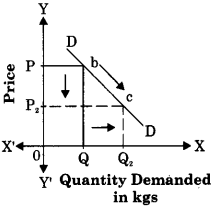In the above diagram, DD is the demand curve which is showing downward movement on the same demand curve from point ‘b’ to point ‘c’ which indicates an expansion of demand.

(2) Contraction of demand : It refers to fall in quantity demanded due to rise in price alone and other factors like tastes, income, population, etc. remaining unchanged. It is shown by a upward movement on the same demand curve.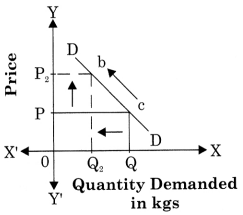In the above diagram, DD is the demand curve which is showing upward movement on the same demand curve from point ‘e’ to point ‘b’ and indicates contraction of demand.

Question 6.
Explain the concept of Giffen Paradox

1. Giffen good are low quality or inferior goods like jowar, palm oil, etc.
2. Demand for low quality goods and inferior goods decrease even if there prices falls.
3. This behaviour of consumer was observed by Sit Robert Giffen in england.
4. Sir Robert Giffen observed that when price of bread (inferior good)declined, people did not buy more of it, because with increased real income they preferred to buy superior goods like meat.
5. In this case, demand curve slopes upward from left to right, showing exception to the law of demand.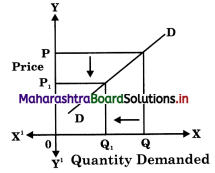4. State with reasons whether you agree or disagree with the following statements:

Question 1.
There are no exceptions to the Law of Demand.
No, I do not agree with this statement.
There are some important cases in which the demand for the commodity is greater when price rises and smaller when price falls. Such cases are called exceptions to the law of Demand. In such case, demand curve slopes upwards from left to right and it has a positive slope.

• Prestige Goods : Rich people buy more expensive goods like gold, diamonds, etc., even when there prices are high to maintain their status.
• Giffen Paradox : Demand for low quality goods and inferior goods decrease even if there prices falls.
According to Sir Robert Giffen when price of bread declined, people did not buy more because of increase in their real income and they prefer to buy superior goods like meat.
• Speculation : People are tend to buy more commodities if they expect prices to rise further. E.g. prices of oil, sugar. etc., are expected to rise before Diwali, so people buy more of these commodities even at higher price.
• Habitual goods : Due to habit of consumption, certain goods like tea is purchased in required quantities even at higher price.
• Ignorance : Sometimes people completely ignore the price of commodity and buy more of that commodity ignoring higher price.
• Price Illusion : Consumer feels that good at higher price are of better quality, therefore demand for such goods are higher even at rise in their prices.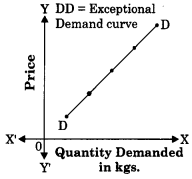Question 2.
Various factors influence the demand for a commodity.
Yes, I agree with this statement.

Meaning of Demand : Demand refers to a desire or want for goods. Desire is the willingness to have some commodity which is backed by willingness and ability to pay.
Definition : According to Benham, “The demand for anything at a given price is the amount of it, which will be bought per unit of time at that price.

Features of Demand :

1. Demand is a relative concept.
2. Demand is essentially expressed with reference to time and price. ?

Determinants of Demand :

1. Price of Complementary Goods : Demand changes with changes in price of complementary goods like car and petrol, etc.
3. Price : Demand for a commodity is mainly influenced by its price. Normally at a higher price the demand is less and at a lower price it is more. Thus, demand varies inversely with price of a commodity.
4. Taste, Habits and Fashions : Habits influence market demand. If people habituated to the consumption of certain goods they will not give up such habits easily. E.g. demand for liquor, cigarettes, etc. Sometimes fashion change attitude and preference of people which in turn changes market demand.
5. Income: Income determines the purchasing power. Rise in income will lead to a rise in demand of a commodity and fall in income will lead to a fall in demand of a commodity.
6. Other Factors : (a) Climatic condition, (b) Changes in technology, (c) Government policy, (d) Customs and traditions, etc.
7. Nature of Product: Under necessary and unavailable circumstances the demand of a commodity will continue to be same irrespective of the corresponding price. E.g. medicine to control blood-pressure.
8. Level of Taxation : There would be increase in price of goods and services due to high rates of taxes which results in a decrease in demand and vice-versa.
9. Expectation about the Future Prices : If the consumer expect a rise in price in the near future they will demand more at present price. Similarly, when they expect price to fall, then they will buy less at present prices.
10. Price of Substitute Goods : Demand for cheaper substitute goods will rise when there is fall in price of such goods. E.g. when sugar price rises, then the demand for jaggery will rise.
11. Size of Population: Demand for commodity depends upon size and composition of population like age structure, gender ratio which influence demand for certain goods. E.g. larger the child population, more will be the demand for toys, chocolates, etc.

Question 3.
The demand curve slopes upwards from left to right.
No, I do not agree with this statement.
OR
Demand curve slopes downward from left to right.
Yes, I agree with this statement.
Reasons justifying downwards sloping demand curve are as follows:

• The law ofDiminishing Marginal Utility: Marginal utility goes on diminishing when there is increase in the stock of commodity and consumer tends to buy more when price falls and vice-versa.
• Income Effects : Whenever there is a fall in price of a commodity, purchasing power of a consumer gets increased, which enables him to buy more of that commodity.
• Substitution Effect : When price of commodity rises consumer tends to buy more of cheaper substitute goods and less of the commodity whose price has increased.
• Multi-purpose Uses : When a commodity can be used for satisfying multiple needs, its demand will rise with a fall in its price and vice-versa.
• New Consumers : When there is fall in price of a commodity, a new consumer class buy the commodity as they can afford it.
Thus total demand for commodity increases with fall in price.

Question 4.
The law of Demand is based on assumptions.
Yes, I agree with this statement.

Assumptions to the Law of Demand:

• Prices of Substitute goods remain constant : The price of substitute goods should remain unchanged, as change in the price will affect the demand for the commodity.
• Prices of Complementary goods remains constant : A change in the price of one good will affect the demand for other, thus the prices of complementary goods should remain unchanged.
• No Expectation about future changes in prices : The consumers do not expect any significance rise or fall in the future prices.
• No change in size of Population : The size of population, gender ratio and age composition are assumed to remain constant. As such changes are sure to affect demand.
• No change in Taxation Policy : The level of direct and indirect tax imposed by the government on the income and goods should remain constant.
• Constant Level of Income : Consumer’s income must remain unchanged because if income increases, consumer may buy more even at a higher price not following the law of demand.
• No Change in Tastes, Habits,
Preference, Fashions, etc. : If the taste changes then the consumers preference will also change which will affect the demand. When commodities are out of fashion, then demand will be low even at a lower price.

Question 5.
Price is the only determinant of demand.
OR
Price is the only factor that affects demand for a commodity.
No, I do not agree with this statement.
There are many other determinants of demand beside price, like –

1. Income: Income determines the purchasing power. Rise in income will lead to a rise in demand of a commodity and fall in income will lead to a fall in demand of a commodity.
2. Price of Substitute Goods : Demand for cheaper substitute goods will rise when there is fall in price of such goods. E.g. when sugar price rises, then the demand for jaggery will rise.
3. Price : Demand for a commodity is mainly influenced by its price. Normally at a higher price the demand is less and at a lower price it is more. Thus, demand varies inversely with price of a commodity.
4. Nature of Product: Under necessary and unavailable circumstances the demand of a commodity will continue to be same irrespective of the corresponding price. E.g. medicine to control blood-pressure.
5. Size of Population: Demand for commodity depends upon size and composition of population like age structure, gender ratio which influence demand for certain goods. E.g. larger the child population, more will be the demand for toys, chocolates, etc.

Question 6.
All desires are not demand.
Yes, I agree with this statement.

• Desire means an individual’s wish to acquire a commodity.
• In economics, demand means desire which is backed by willingness and ability to pay.
• Symbolically, Demand = Desire + Ability to pay + Willingness to pay
• All desires may not be backed by ability to pay and willingness to pay.
• Therefore, all desires are not demand.Question 7.
Population is the only determinant or factors of demand. (Mar. ‘15)
No, I do not agree with this statement.

Determinants of Demand :

1. Price of Complementary Goods : Demand changes with changes in price of complementary goods like car and petrol, etc.
3. Price : Demand for a commodity is mainly influenced by its price. Normally at a higher price the demand is less and at a lower price it is more. Thus, demand varies inversely with price of a commodity.
4. Taste, Habits and Fashions : Habits influence market demand. If people habituated to the consumption of certain goods they will not give up such habits easily. E.g. demand for liquor, cigarettes, etc. Sometimes fashion change attitude and preference of people which in turn changes market demand.
5. Income: Income determines the purchasing power. Rise in income will lead to a rise in demand of a commodity and fall in income will lead to a fall in demand of a commodity.
6. Other Factors : (a) Climatic condition, (b) Changes in technology, (c) Government policy, (d) Customs and traditions, etc.

Question 8.
There are many types of demand.
Yes, I agree with this statement.

1. Direct Demand : When a commodity satisfies the want of a consumer directly, it is said to have direct demand. Demand for consumer goods like food, clothes, sugar, vegetables, milk, etc.
2. Indirect Demand : It refers to demand for goods which are required for further production. It is also called as derived demand. Demand for factors of production like land, labour, capital, etc.
3. Joint or Complementary Demand : When two or more goods are demanded at a same time to satisfy single want, it is known as Joint or Complementary Demand. E.g. car and fuel, pen and ink, mobile phone and sim card, etc.
4. Composite Demand : When one commodity can be utilize for several needs or multiple uses, it is known as composite demand. E.g. electricity, steel, coal, etc.
5. Competitive Demand : It is demand for those goods which are substitute for each other. E.g. tea or coffee, sugar or jaggery, pepsi or Thumsup, etc.

Question 1.
State and explain the law of Demand with assumptions.
(A) Introduction : The law of demand is one of the important law of consumption which explain the functional relationship between price and quantity demanded of a commodity. Prof. Alfred Marshall in his book ‘Principle of Economics’ which was published in 1890, has explained the consumer’s behaviour as follows:

(B) Statement of the Law : According to Prof. Alfred Marshall, “Other things being equal, higher the price of a commodity, smaller is the quantity demanded and lower the price of a commodity, larger is the quantity demanded.
In other words, other things remaining constant, demand varies inversely with price. Marshall’s law of demand describes the functional relationship between demand and price. It can be presented as:
Dx = f(Px)
where D = Demand for Commodity
x = Commodity
f = function
Px = Price of a commodity
(C) Assumption :

• Prices of Substitute goods remain constant : The price of substitute goods should remain unchanged, as change in the price will affect the demand for the commodity.
• Prices of Complementary goods s remains constant : A change in the price j of one good will affect the demand for other,  thus the prices of complementary goods  should remain unchanged.
• No Expectation about future changes jj in prices: The consumers do not expect any \ significance rise or fall in the future prices.
• No change in Taxation Policy : The level of direct and indirect tax imposed by the government on the income and goods should remain constant.
• Constant Level of Income : Consumer’s income must remain unchanged because if income increases, consumer may buy more even at a higher price not following the law of demand.
• No Change in Tastes, Habits, Preference, Fashions, etc. : If the taste changes then the consumers preference will also change which will affect the demand. When commodities are out of fashion, then demand will be low even at a lower price.

(D) Explanation of the law of Demand :
The law of demand is explained with the help of the following demand schedule and diagram:
Demand Schedule

 Price of Commodity ‘X’ (in Rs.) Quantity Demanded of Commodity ‘X’ (in kgs) 50 1 40 2 30 3 20 4 10 5

From the above demand schedule we observe that at higher price of ₹ 50 per kg, quantity demanded is 1 kg. When price fall from ₹ 50 to ₹ 40, quantity demanded rises from 1 kg to 2 kg. Similarly, at price ₹ 30 quantity demanded is 3kg and when price falls from ₹ 20 to ₹ 10 quantity demanded rises from 4 kg to 5 kg. This shows an inverse relationship between price and demand.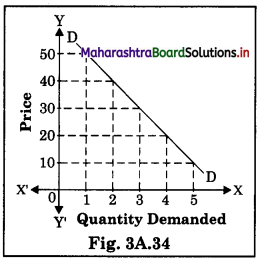In the above diagram X-axis represent quantity demanded and Y-axis represent the price of the commodity. The demand curve DD slopes downwards from left to right ] showing an inverse relationship between price and demand. It has a negative slope.